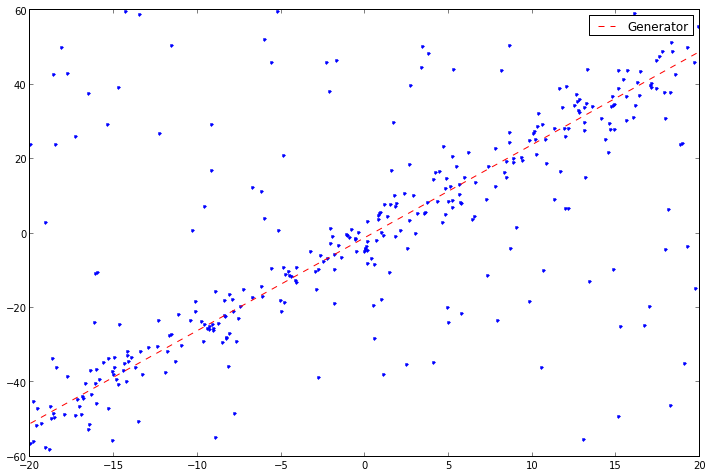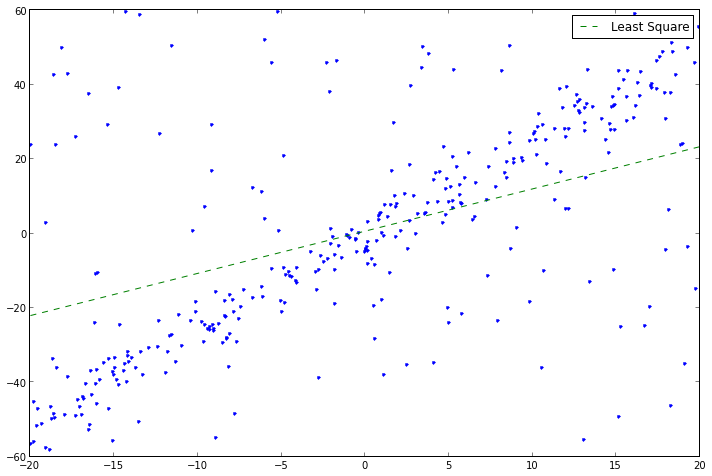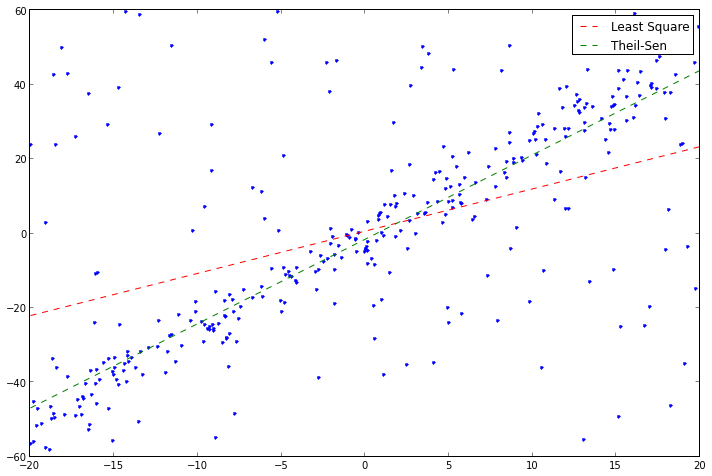# Autotelic Computing

## Thursday, December 12, 2013

### Robustified Linear Regression

Let's revisit our toy linear regression problem, and in particular, look at what happens when the data contains a lot of outliers.

In :
rcParams['figure.figsize'] = 12, 8

domain = [-20, 20]

def dataset(n, slope, intercept):
x = random.uniform(domain, domain, n)
noise = random.normal(0, 5, n)
y = slope * x + intercept + noise
return column_stack([x, y])

points = dataset(250, 2.5, -1)

extra_noise = column_stack([
random.uniform(domain, domain, 100),
random.uniform(-60, 60, 100)
])
points = vstack([points, extra_noise])

plot(points[:,0], points[:,1], '.')
plot(linspace(domain, domain),
-1 + 2.5 * linspace(domain, domain),
'r--', label='Generator', linewidth=1)
legend();If we reuse our least square gradient descent method, we see that it does not perform as well anymore.

In :
def least_square_linear_regression(points, initial_theta, alpha=0.00001, stop=1e-5):
x, y = points[:,0], points[:,1]
theta = initial_theta
prev_mse = float('inf')
while True:
theta = theta.copy()
h = theta + theta * x
theta -= alpha * sum(h - y)
theta -= alpha * sum((h - y) * x)
mse = sum((h - y) ** 2) / len(points)
if mse - prev_mse < stop: break
prev_mse = mse
return theta

initial_theta = random.sample(2)
ls_theta = least_square_linear_regression(points, initial_theta)
print ls_theta
plot(points[:,0], points[:,1], '.')
plot(linspace(domain, domain),
ls_theta + ls_theta * linspace(domain, domain),
'g--', label='Least Square')
legend();

[ 0.67349646  1.13530362]To mitigate the problem, we can try a completely different way of searching for the right set of parameters, not based on either least squares or gradient descent: the Theil-Sen estimator. This non-parametric method is very simple: set the slope $m$ of the model as the median of the slopes resulting from each pair of training points (i.e. $(y_j − y_i)/(x_j − x_i))$ for every pair $(i, j)$). Once set, find the intercept $b$ in a similar way, as the median of $y_i − m x_i$ for every $i$. The results show that the solution obtained is really more robust, as it's less sensitive to outliers. The naive version of this algorithm, which is used here, while nice because it holds in two lines of Python, wouldn't work very well however on a bigger dataset, because of its quadratic running time (due to the fact that we're enumerating the ${{n}\choose{2}}$ combinations). There are however more efficient algorithms, based on sorting, that can do it in $O(n \log n)$.

In :
from itertools import combinations

# WARNING! Naive code below!
def theil_sen_linear_regression(points):
m = median([(q - p) / (q - p)
for p, q in combinations(points, 2)])
b = median([p - m * p for p in points])
return (b, m)

ts_theta = theil_sen_linear_regression(points)
print ts_theta

plot(points[:,0], points[:,1], '.')
plot(linspace(domain, domain),
ls_theta + ls_theta * linspace(domain, domain),
'r--', label='Least Square')
plot(linspace(domain, domain),
ts_theta + ts_theta * linspace(domain, domain),
'g--', label='Theil-Sen')
legend();


(-1.498047457480939, 2.2686431432094376)